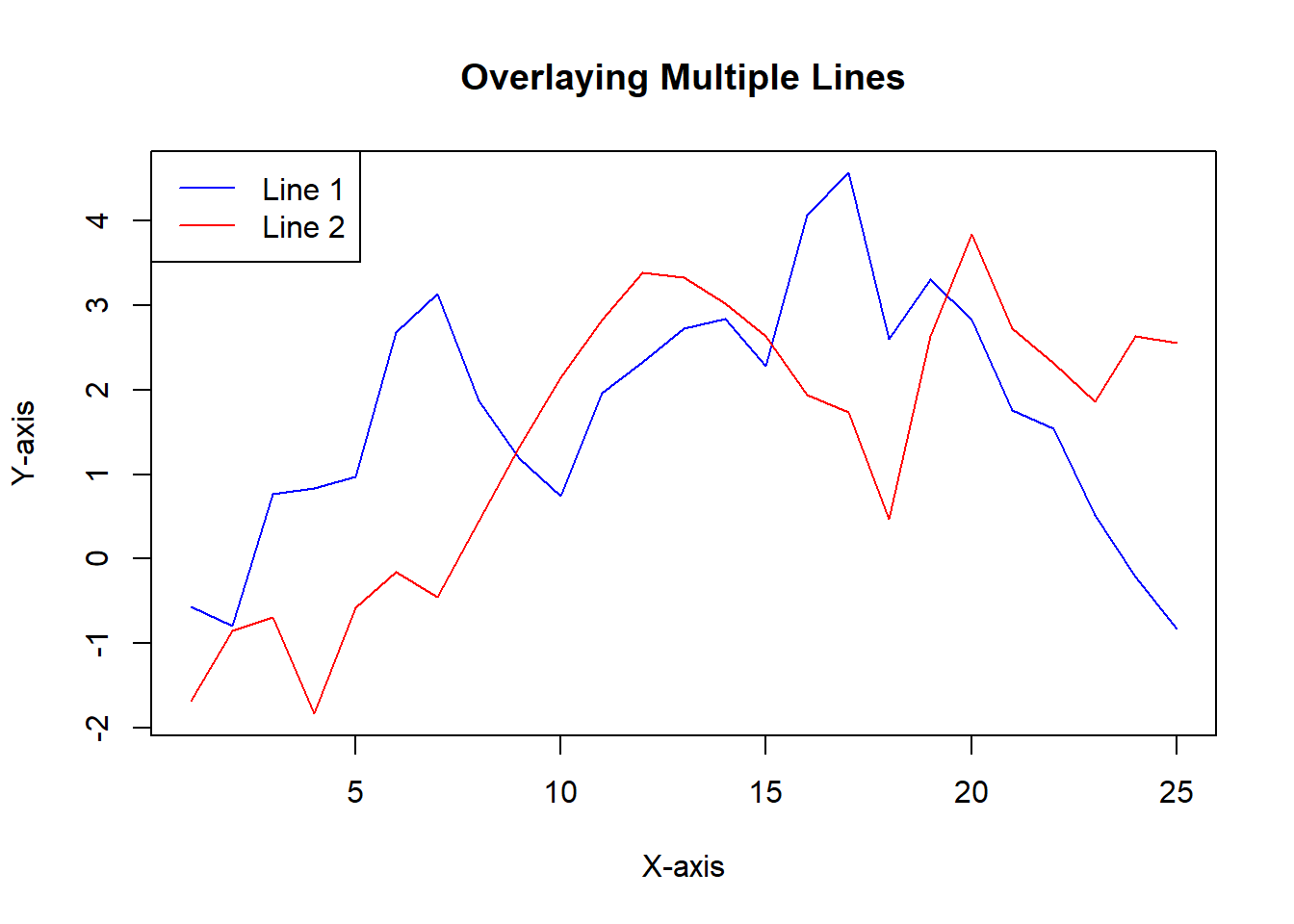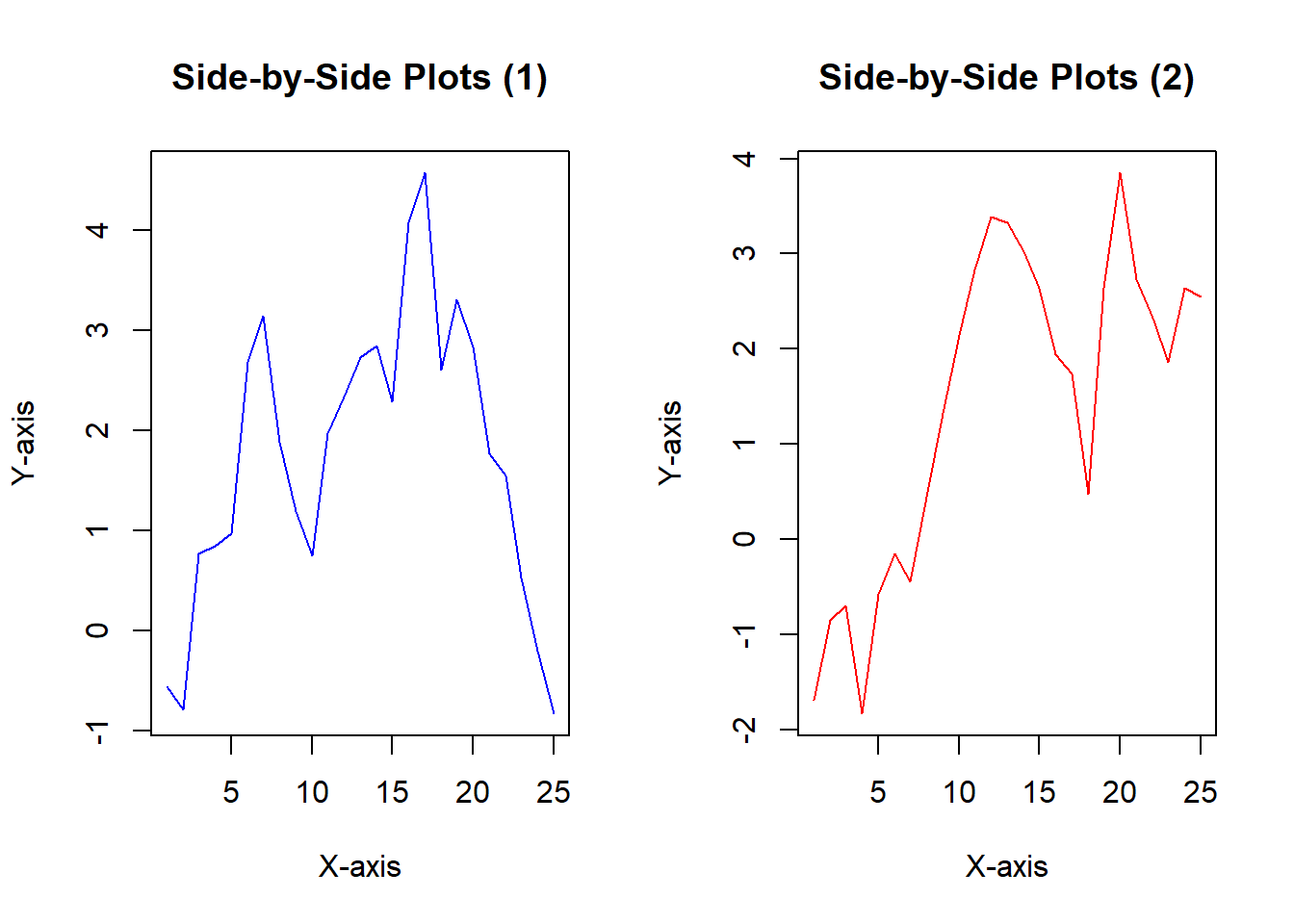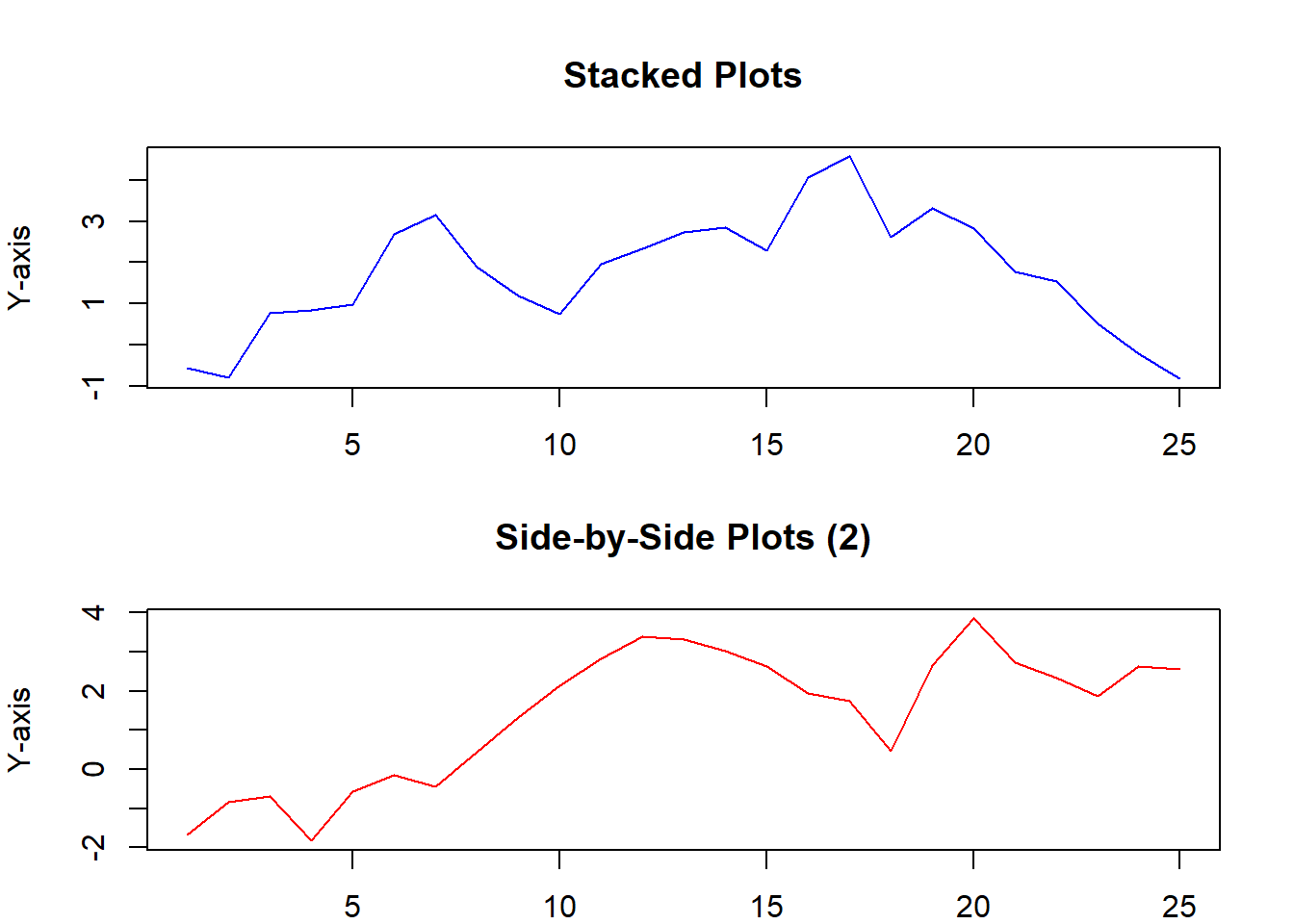# How to Plot Multiple Plots on the Same Graph in R

rtip
viz
Author

Steven P. Sanderson II, MPH

Published

September 13, 2023

# Introduction

Data visualization is a crucial aspect of data analysis. In R, the flexibility and power of its plotting capabilities allow you to create compelling visualizations. One common scenario is the need to display multiple plots on the same graph. In this blog post, we’ll explore three different approaches to achieve this using the same dataset. We’ll use the `set.seed(123)` and generate data with `x` and `y` equal to `cumsum(rnorm(25))` for consistency across examples.

# Examples

## Example 1: Overlaying Multiple Lines on the Same Graph

In this example, we will overlay two lines on the same graph. This is a great way to compare trends between two variables in a single plot.

``````# Set the seed for reproducibility
set.seed(123)

# Generate the data
x <- 1:25
y1 <- cumsum(rnorm(25))
y2 <- cumsum(rnorm(25))

# Create the plot
plot(x, y1, type = 'l', col = 'blue', ylim = c(min(y1, y2), max(y1, y2)),
xlab = 'X-axis', ylab = 'Y-axis', main = 'Overlaying Multiple Lines')
lines(x, y2, col = 'red')
legend('topleft', legend = c('Line 1', 'Line 2'), col = c('blue', 'red'), lty = 1)``````In this code, we first generate the data for `y1` and `y2`. Then, we use the `plot()` function to create a plot of `y1`. We specify `type = 'l'` to create a line plot and set the color to blue. Next, we use the `lines()` function to overlay `y2` on the same plot with a red line. Finally, we add a legend to distinguish the two lines.

## Example 2: Side-by-Side Plots

Sometimes, you might want to display multiple plots side by side to compare different variables. We can achieve this using the `par()` function and layout options.

``````# Create a side-by-side layout
par(mfrow = c(1, 2))

# Create the first plot
plot(x, y1, type = 'l', col = 'blue',
xlab = 'X-axis', ylab = 'Y-axis', main = 'Side-by-Side Plots (1)')

# Create the second plot
plot(x, y2, type = 'l', col = 'red',
xlab = 'X-axis', ylab = 'Y-axis', main = 'Side-by-Side Plots (2)')````````````# Reset Par
par(mfrow = c(1, 1))``````

In this example, we use `par(mfrow = c(1, 2))` to set up a side-by-side layout. Then, we create two separate plots for `y1` and `y2`.

## Example 3: Stacked Plots

Stacked plots are useful when you want to compare the overall trend while preserving the individual patterns of different variables. Here, we stack two line plots on top of each other.

``````par(mfrow = c(2, 1), mar = c(2, 4, 4, 2))

# Create the first plot
plot(x, y1, type = 'l', col = 'blue',
xlab = 'X-axis', ylab = 'Y-axis', main = 'Stacked Plots')

# Create the second plot
plot(x, y2, type = 'l', col = 'red',
xlab = 'X-axis', ylab = 'Y-axis', main = 'Side-by-Side Plots (2)')````````par(mfrow = c(1, 1))``

The first line of code, `par(mfrow = c(2, 1), mar = c(2, 4, 4, 2))`, tells R to create a 2x1 (two rows, one column) plot with margins of 2, 4, 4, and 2. This means that the two plots will be stacked on top of each other.

The next line of code, `plot(x, y1, type = 'l', col = 'blue', xlab = 'X-axis', ylab = 'Y-axis', main = 'Stacked Plots')`, create the first plot. The `plot()` function creates a plot of the data in the vectors x and y1. The `type = 'l'` argument tells R to create a line plot, the col = ‘blue’ argument tells R to use blue color for the line, and the other arguments set the labels for the axes and the title of the plot.

The fourth line of code, `plot(x, y2, type = 'l', col = 'red', xlab = 'X-axis', ylab = 'Y-axis', main = 'Side-by-Side Plots (2)')`, create the second plot. This plot is similar to the first plot, except that the line is red.

The last line of code, `par(mfrow = c(1, 1))`, resets the plot to a single plot.

In summary, this code creates two line plots, one stacked on top of the other. The first plot uses blue lines and the second plot uses red lines. The plots are labeled and titled appropriately.

# Conclusion

In this blog post, we explored three different techniques for plotting multiple plots on the same graph in R. Whether you need to overlay lines, display plots side by side, or stack them, R offers powerful tools to visualize your data effectively. Try these examples with your own data to harness the full potential of R’s plotting capabilities and create informative visualizations for your analyses. Happy plotting!